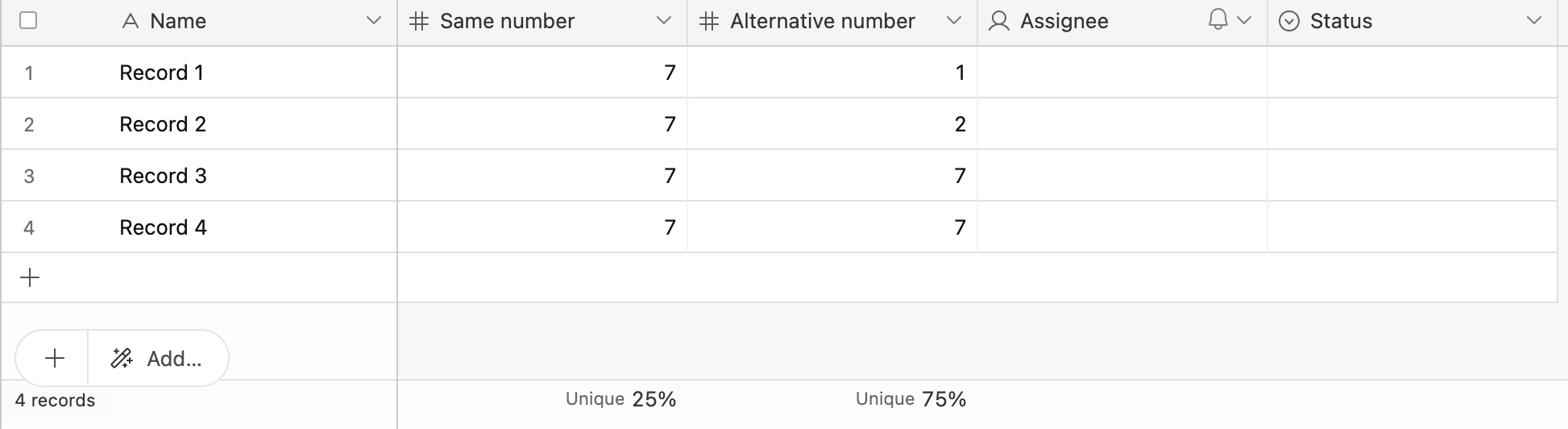• English
• Account
• API
• Integrations
• Enterprise
• Resources
Using the summary bar in Airtable views
• 12 Jun 2023
• Dark
Light

# Using the summary bar in Airtable views

• Dark
Light

Article Summary

Learn about the summary bar, an Airtable base metadata feature that provides different types of summarizations of all the cell values in a given field.

Note

The summary bar is a great tool that can be used to quickly understand and calculate values for a set of records. However, the summary bar can't be referenced by Airtable records. Summary values are a type of metadata not currently accessible by record-level formulas.

## Introduction

 Plan availability All plan types Permissions All users with base access Platform(s) Web/Browser, Mac app, Windows app, and mobile apps Related reading Timeline view - Summary bar

## Summary bar overview

Where to find the summary bar

The summary bar is located at the bottom of some view types within a table. Certain field types will offer different summary options. Those differences are covered in more depth below.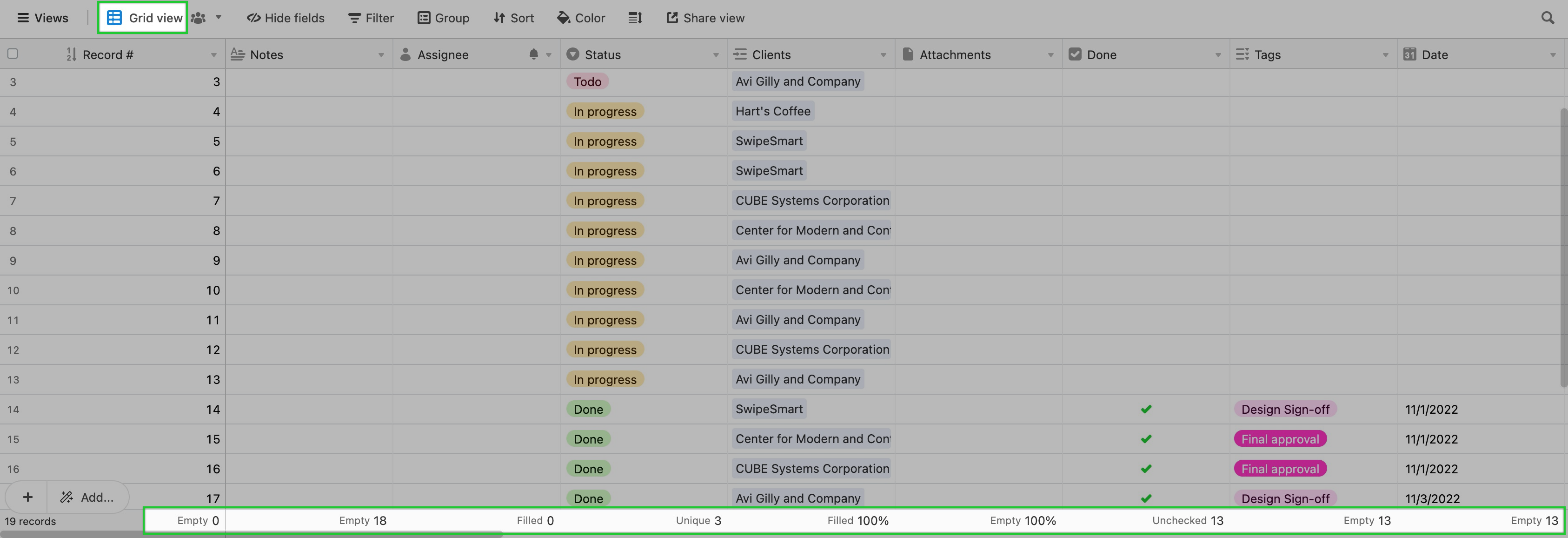Summary bar functions

By default, the summary function for each numeric field is Sum, and the summary function for non-numeric fields is blank. To select a different summarization function for a specific field, click on the summary bar below that field. This will bring up a menu of different summary functions. The types of summary functions available will depend on the type of field.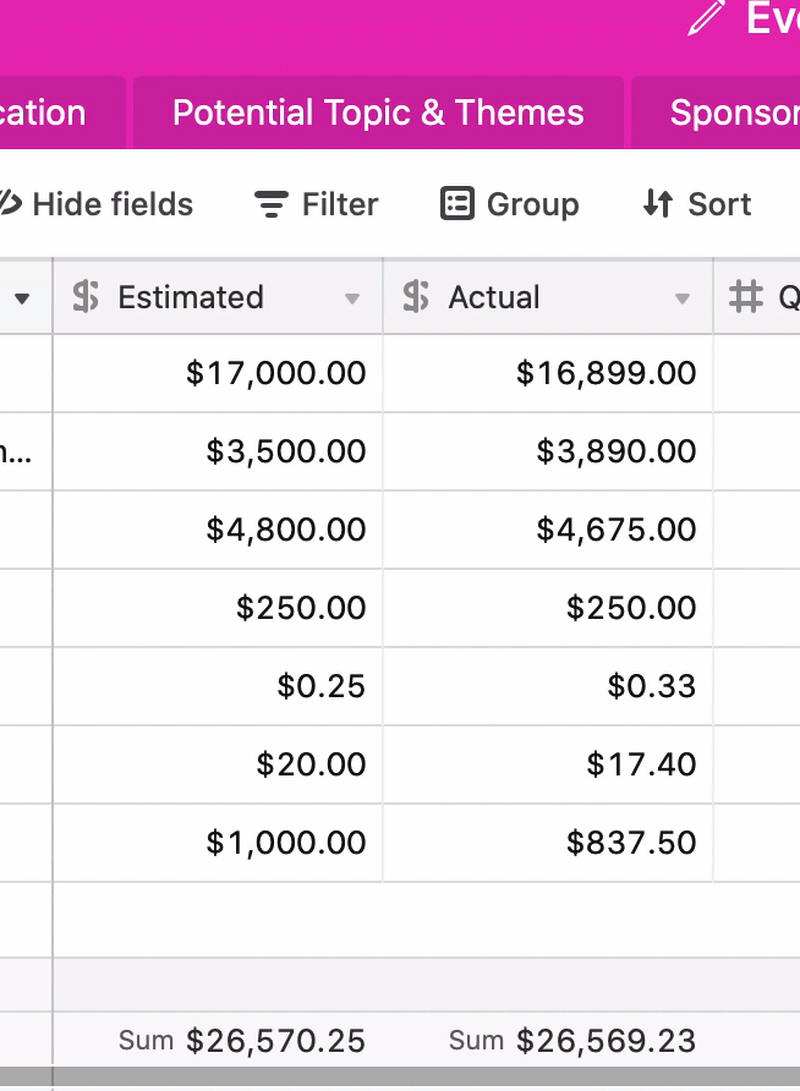Summarizing a subset of records

If you select a range of rows or cells, the summarization will only include cells that are in that selection range.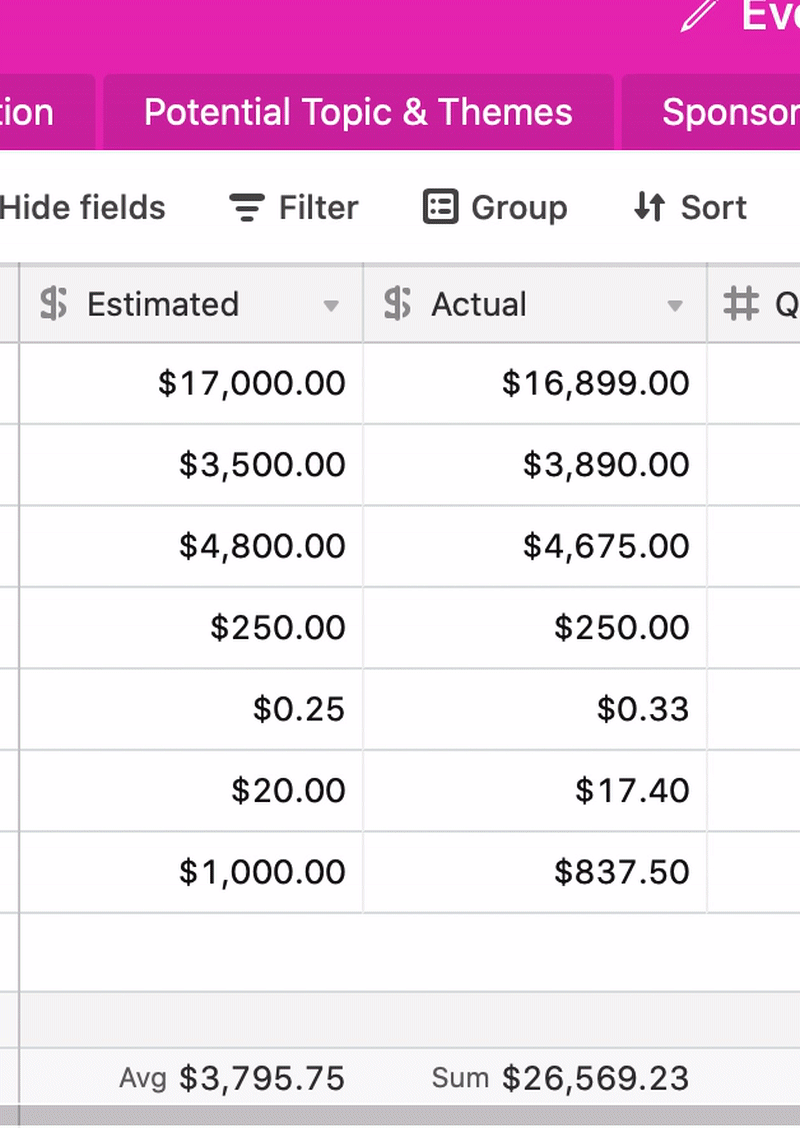Grouped summaries

If the records in your view are grouped then your summary bar will automatically show a separate summary for each group, as well as the total summary for the field as a whole: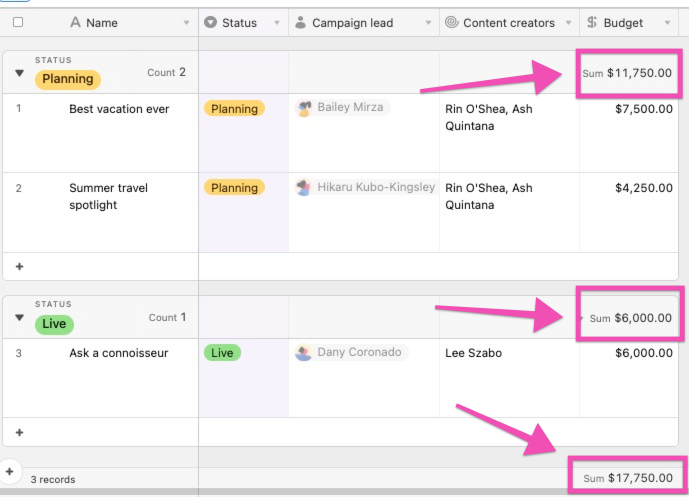## General summary functions

Non-calculated fields

Certain summary functions are available in all non-calculated fields (i.e. not formula, rollup, or lookup).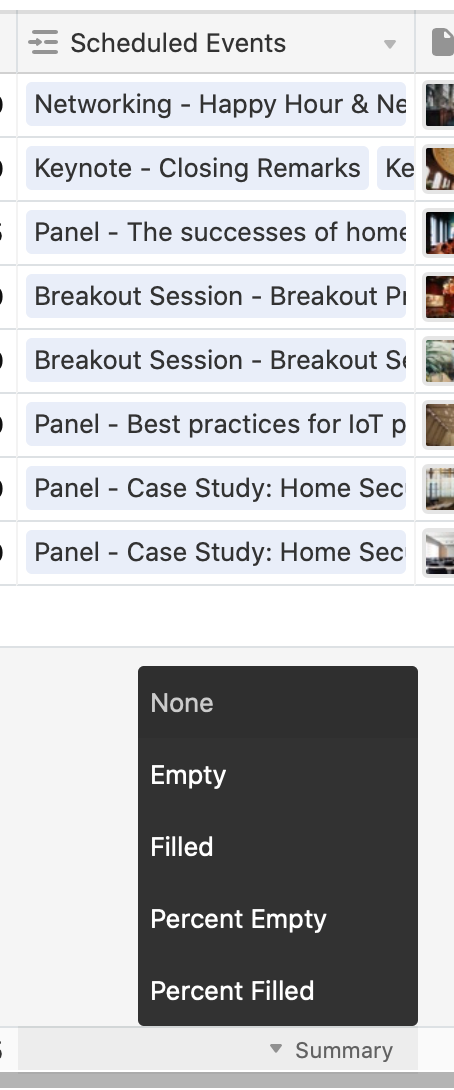They are, as follows:

• Empty
• Filled
• Percent Empty
• Percent Filled
Checkbox fields

For checkbox fields, the summary functions will display slightly differently, as Unchecked, Checked, Percent Unchecked, and Percent Checked.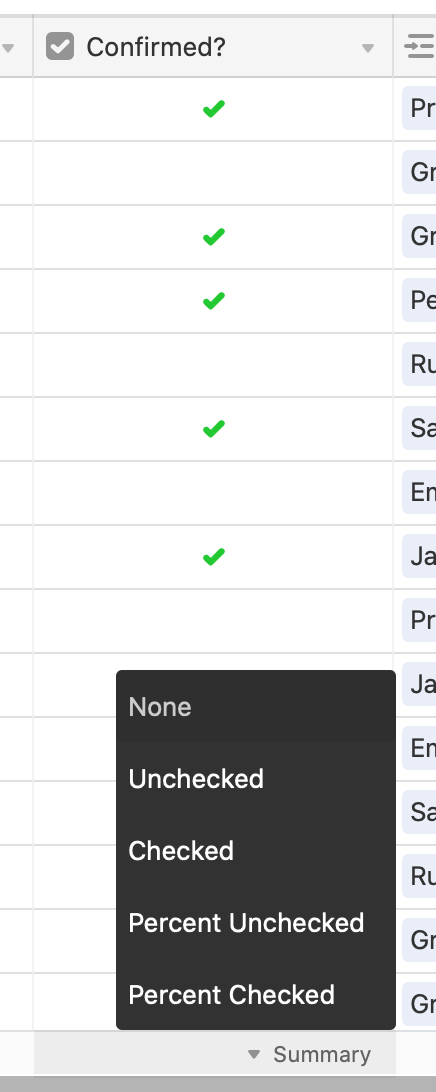Unique summaries

Many field types will also include Unique and Percent Unique. These functions allow you to calculate the absolute number of unique values present in a field, and the percentage of cell values that are unique in a field, respectively.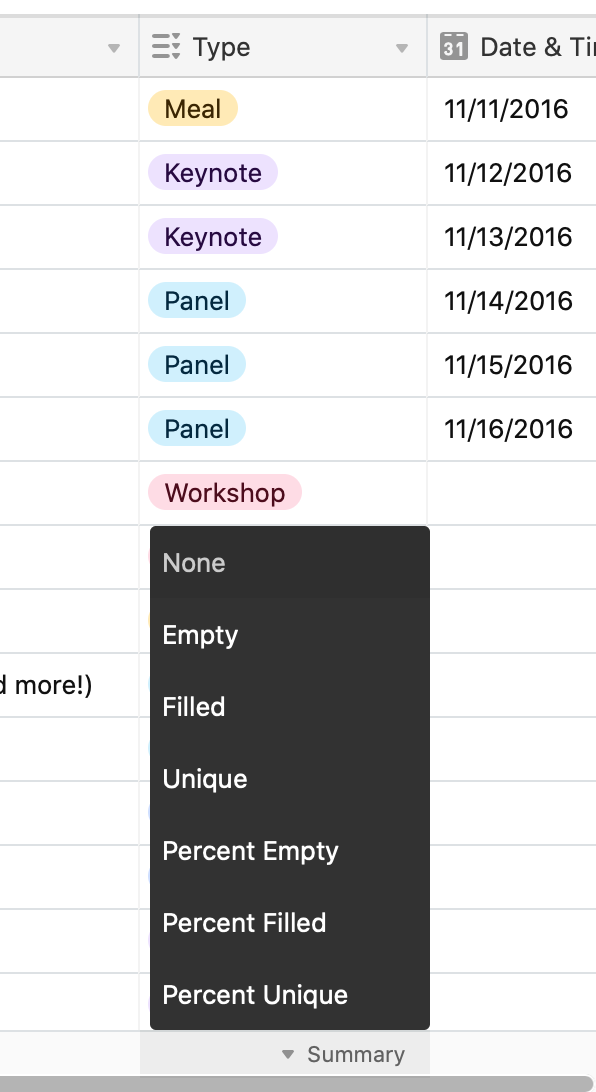## Special functions for numeric fields

Special functions for numeric fields

Certain functions will only appear in numeric fields (i.e. number, currency, percent, and autonumber).

• Sum
• Average
• Histogram
• Min
• Max
• Range
• Median
• Standard Deviation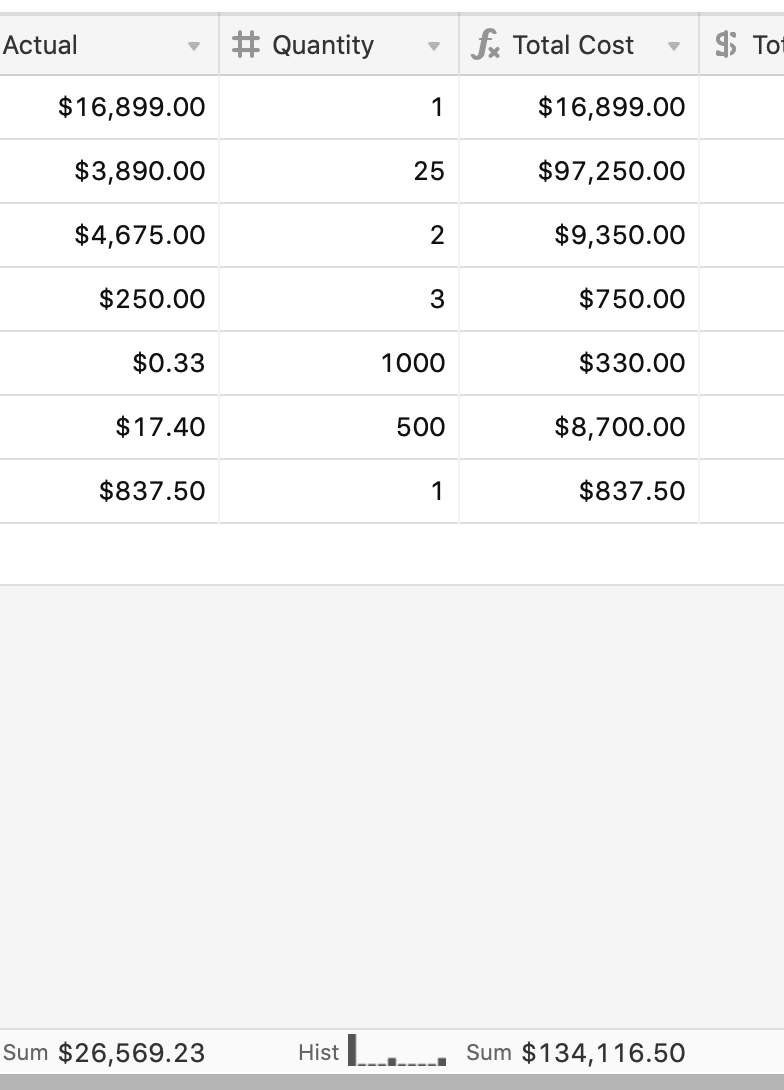Special functions for date fields

Certain functions will only appear in date fields. These are, as follows:

• Earliest Date
• Latest Date
• Date Range (days)
• Date Range (months)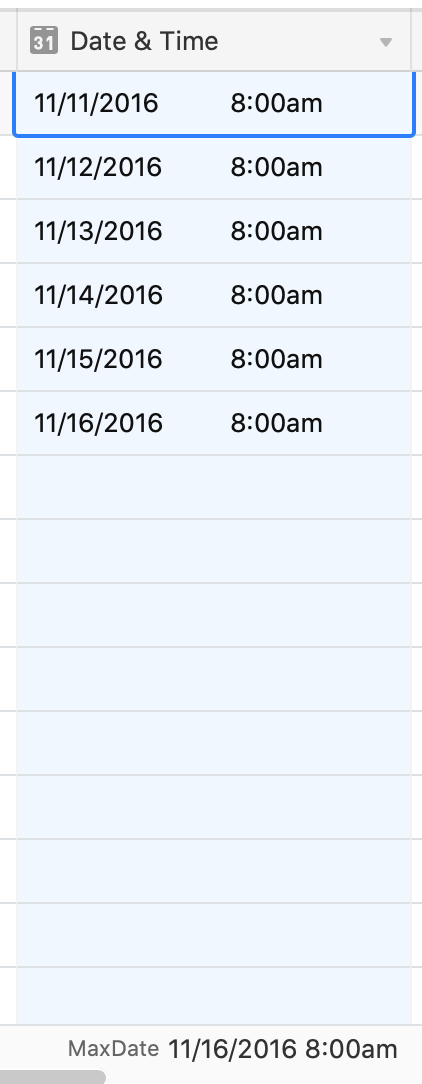Special function for attachment fields

A special summary function for an attachment field allows you to see the total size of the file attachments in the attachment field, in bytes.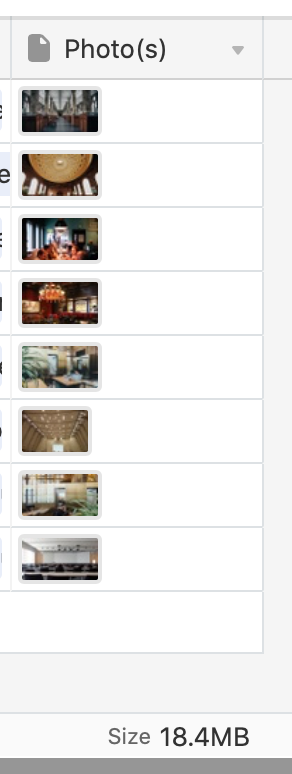## FAQs

How is the Percent unique function calculated?

The Percent unique function calculates the ratio of cells containing unique values in a field. It counts the number of distinct values and divides that number by the total count of cells in the field. This provides a useful way to understand the variety and distribution of data within a field in your Airtable base, even when values are repeated.

Example:

• Let's say you have four records. Each record contains a number field and each cell within that field contains the number 7.
• The Percent unique summary function identifies that there is 1 unique value here, which is 7. It calculates the Percent unique as 1 (unique value) divided by 4 (total cells/records), giving 0.25, or 25%.
• Alternatively, if you have four cells containing multiple values, like this: [1, 2, 7, 7], the function identifies 3 distinct or unique values, which are 1, 2, and 7. It calculates the Percent unique as 3 (unique values) divided by 4 (total cells/records), giving 0.75, or 75%.